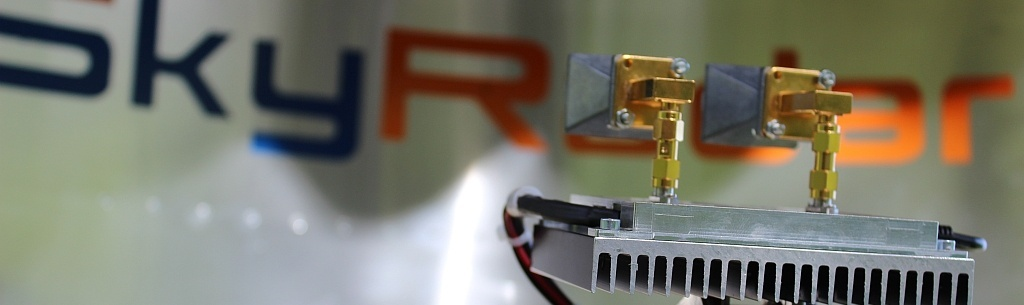Free Media » Trade Shows » FOURIER TRANSFORM METHODS IN GEOPHYSICS

FOURIER TRANSFORM METHODS IN GEOPHYSICS

David Sandwell introduces into the use of Fourier transform in geophysics such as image processing,time series analysis, and antenna design. He focusses “on the use of fourier transforms for solving linear partial differential equations (PDE). Some examples include: Poisson’s equation for problems in gravity and magnetics; the biharmonic equation for problems in linear visco-elasticity; and the diffusion equation for problems in heat conduction.”